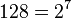# Cyclic group:Z128

View a complete list of particular groups (this is a very huge list!)[SHOW MORE]

## Definition

This group is defined as the cyclic group of order$128 = 2^7$, denoted$C_{128}$,$\mathbb{Z}_{128}$, or$\mathbb{Z}/128\mathbb{Z}$.

## Arithmetic functions

Want to compare and contrast arithmetic function values with other groups of the same order? Check out groups of order 128#Arithmetic functions
Function Value Similar groups Explanation for function value
underlying prime of p-group 2
order (number of elements, equivalently, cardinality or size of underlying set) 128 groups with same order
prime-base logarithm of order 7 groups with same prime-base logarithm of order
max-length of a group 7 max-length of a group equals prime-base logarithm of order for group of prime power order
chief length 7 chief length equals prime-base logarithm of order for group of prime power order
composition length 7 composition length equals prime-base logarithm of order for group of prime power order
exponent of a group 128 groups with same order and exponent of a group | groups with same prime-base logarithm of order and exponent of a group | groups with same exponent of a group
prime-base logarithm of exponent 7 groups with same order and prime-base logarithm of exponent | groups with same prime-base logarithm of order and prime-base logarithm of exponent | groups with same prime-base logarithm of exponent
Frattini length 7 groups with same order and Frattini length | groups with same prime-base logarithm of order and Frattini length | groups with same Frattini length Frattini length equals prime-base logarithm of exponent for abelian group of prime power order
minimum size of generating set 1 groups with same order and minimum size of generating set | groups with same prime-base logarithm of order and minimum size of generating set | groups with same minimum size of generating set
subgroup rank of a group 1 groups with same order and subgroup rank of a group | groups with same prime-base logarithm of order and subgroup rank of a group | groups with same subgroup rank of a group same as minimum size of generating set since it is an abelian group of prime power order
rank of a p-group 1 groups with same order and rank of a p-group | groups with same prime-base logarithm of order and rank of a p-group | groups with same rank of a p-group same as minimum size of generating set since it is an abelian group of prime power order
normal rank of a p-group 1 groups with same order and normal rank of a p-group | groups with same prime-base logarithm of order and normal rank of a p-group | groups with same normal rank of a p-group same as minimum size of generating set since it is an abelian group of prime power order
characteristic rank of a p-group 1 groups with same order and characteristic rank of a p-group | groups with same prime-base logarithm of order and characteristic rank of a p-group | groups with same characteristic rank of a p-group same as minimum size of generating set since it is an abelian group of prime power order
nilpotency class 1 The group is a nontrivial abelian group
derived length 1 The group is a nontrivial abelian group
Fitting length 1 The group is a nontrivial abelian group

-

## GAP implementation

### Group ID

This finite group has order 128 and has ID 1 among the groups of order 128 in GAP's SmallGroup library. For context, there are groups of order 128. It can thus be defined using GAP's SmallGroup function as:

SmallGroup(128,1)

For instance, we can use the following assignment in GAP to create the group and name it$G$:

gap> G := SmallGroup(128,1);

Conversely, to check whether a given group$G$ is in fact the group we want, we can use GAP's IdGroup function:

IdGroup(G) = [128,1]

or just do:

IdGroup(G)

to have GAP output the group ID, that we can then compare to what we want.